# Bokeh中独特的数据类型简介： ColumnDataSource

Bokeh

Bokeh中独特的数据类型简介： ColumnDataSource

• window 7 系统
• python 3.6
• Jupyter Notebook
• bokeh 0.13.0

（1）直接提供数据

（2）通过 ColumnDataSource 来提供数据

# 1 直接提供数据

from bokeh.plotting import figure, output_notebook, show
from bokeh.layouts import gridplot
from bokeh.models import ColumnDataSource
import numpy as np
import pandas as pd

output_notebook()


np.random.seed(15)

x=np.random.randint(1,20,size=6)
y=np.random.randint(20,50,size=6)

print(x)
print(y)

p = figure(plot_width=300, plot_height=300)
p.circle(x, y,size=y)

show(p)

[ 9 13  6  1  8 12]
[41 42 35 49 37 33]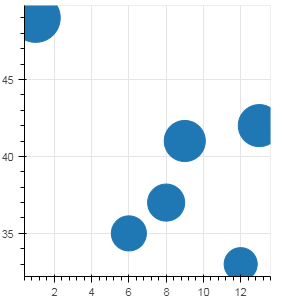# 2 通过 ColumnDataSource 来提供数据

ColumnDataSource 是 Bokeh 中一种重要的数据形式，ColumnDataSource() 方法有一个参数为 “data”， “data”主要有以下三种类型：

（1）data 为字典

（2）data 为 Pandas 的 DataFrame

（3）data 为 Pandas 的 DataFrame 的 groupby 对象

## 2.1 data 为字典

data 的表现形式是一个字典的形式， 一般情况下， 字典的 key 值是一个字符串，代表列名称， 而 value则是 list形式 或者 numpy的 array 形式。 演示如下：

data = {'x_values': [1, 2, 9, 4, 5],
'y_values': [6, 7, 2, 3, 6]}

source = ColumnDataSource(data=data)
source

ColumnDataSource(
id = '0d38f463-7107-49e7-9263-7b5395afc00a', …)
type(source)

bokeh.models.sources.ColumnDataSource


data = {'x_values': [1, 2, 9, 4, 5],
'y_values': [6, 7, 2, 3, 6]}

source = ColumnDataSource(data=data)

p = figure(plot_width=300, plot_height=300)
p.circle(x='x_values', y='y_values', source=source, size=20)
show(p)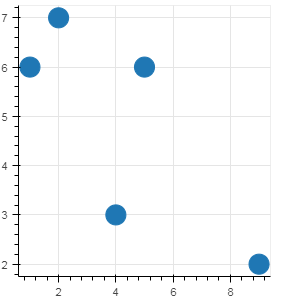## 2.2 data 为 pandas 的 DataFrame

ColumnDataSource （简称为 CDS） 的 data 参数，也可以是 pandas 的 DataFrame。 当CDS的参数是 DataFrame 时，参数中可以直接用 DataFrame 的列名称，索引名称，也可以直接用DataFrame 已有的名称， 如果没有索引名称，默认情况下， 索引名称 用 “index” 就可以。

data = {'x_column': [1, 2, 9, 4, 5, 8],
'y_column': [6, 7, 2, 3, 6, 2]}

df = pd.DataFrame(data=data)
df

x_column y_column
0 1 6
1 2 7
2 9 2
3 4 3
4 5 6
5 8 2
source_df = ColumnDataSource(df)

p = figure(plot_width=300, plot_height=300)
p.circle(x='x_column', y='y_column', source=source_df, size=15)
show(p)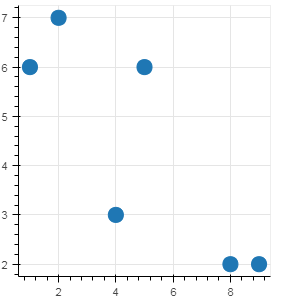source_df = ColumnDataSource(df)

p = figure(plot_width=300, plot_height=300)

# 使用 “index” 作为 DataFrame 的默认索引名称
p.circle(x= 'index', y='y_column', source=source_df, size=15)
show(p)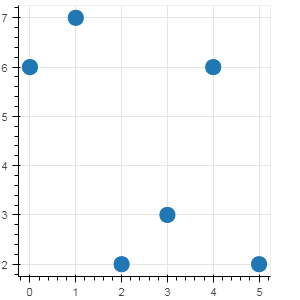## 2.3 data 为 pandas 的 DataFrame 的 groupby 对象

ColumnDataSource （简称为 CDS） 的 data 参数，还也可以是 pandas 的 DataFrame 的 groupby 对象。

dates = pd.date_range('20180101', periods=360)

df = pd.DataFrame(np.random.randn(360,2), index=dates, columns=list('AB'))

df['C'] = ['Good', 'Bad', 'Common', 'Good','Good']*72
df['month'] = df.index.month

df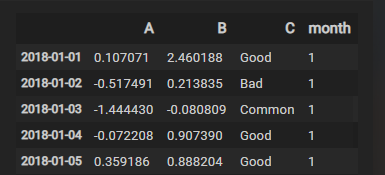g = df.groupby('month')

g.describe()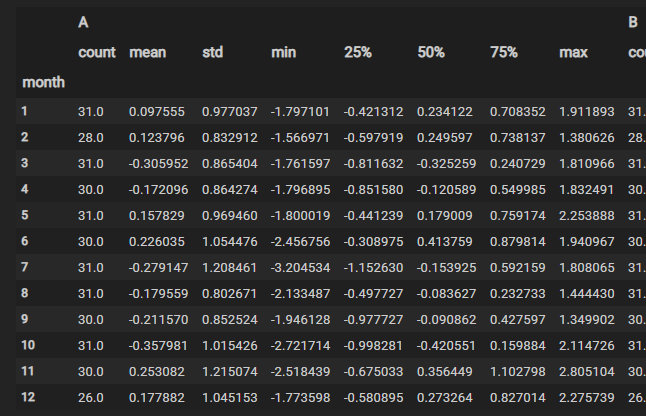source_g = ColumnDataSource(g)
p = figure(plot_width=400, plot_height=300)
p.vbar(x='month', width=0.3, bottom=0, top='A_mean',source=source_g)
show(p)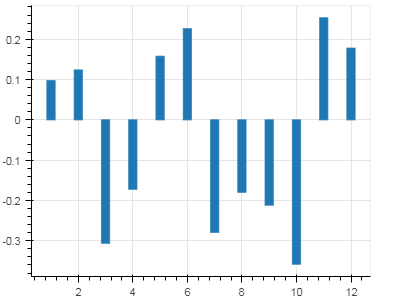# 3 小结